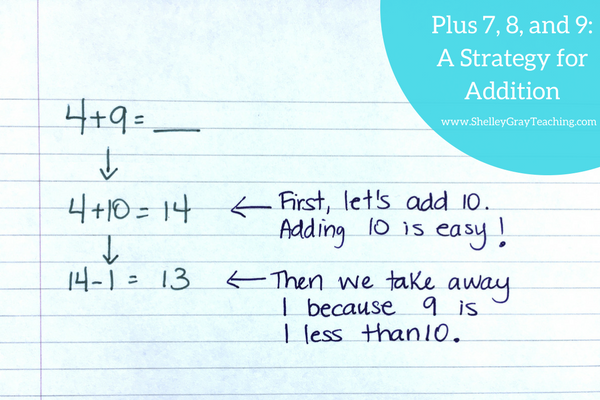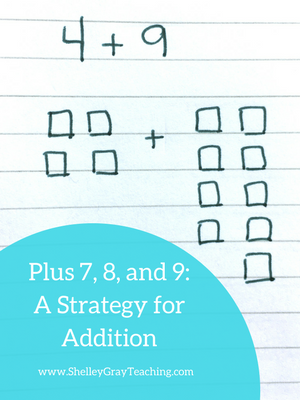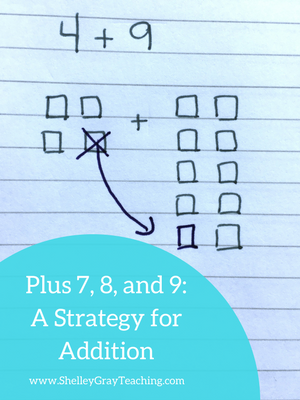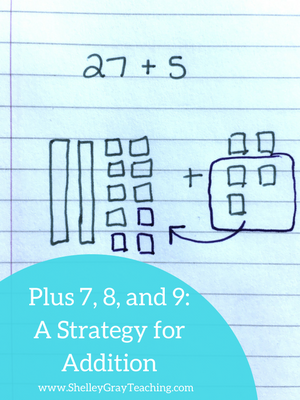# Plus 7, 8, and 9: An Addition Strategy

Depending on the grade level that you teach, you will handle adding plus 7, 8, and 9 a bit different in your classroom. There are two major concepts to reinforce when it comes to adding 7, 8, and 9 to a number.

ADDING 10 AND THEN TAKING 1, 2, OR 3 AWAY

MAKING A 10

Let’s discuss both of these concepts.

ADDING 10 AND THEN TAKING AWAY

This is a strategy that you will focus on in younger grades, particularly first and second grade. It can still be reinforced in older grades as well. When our students are faced with an equation like 4+9=___, we encourage them to first think, “4+10=14 and then we can take one away to make 13.”Similarly, to add 8 to a number, we can add 10 first and then take away 2, since 8 is 2 less than 10. So for the equation 7+8=___, we can think, “7+10=17, and then we can take two away to make 15.”MAKING A 10

When students get a bit older (3rd and 4th grades), we can extend this knowledge. Now we tend to focus even more on flexible thinking – the big goal of mental math.

Now, when our students are faced with an equation such as 4+9=___, we can encourage them to think, “I can take 1 away from the 4 and give it to the 9 to make 10, and then add the remaining 3 to make 13.”To add 8+7, we can think, “I will take 2 from the 7 and give it to the 8 to make a 10, and then add the remaining 5 to make 15.”

This concept can be extended to bigger numbers as well – numbers that end in 7, 8, or 9. For example, to add 27+5, take 3 away from the 5 and give it to the 27 to make 30, and then add the remaining 2 to make 32.”MORE THAN JUST AN ISOLATED STRATEGY

One of the main conflicting opinions with strategies like this is that they are impractical and take too long. However, we must remember that the goal of mental math is more than simply solving an equation. The big goal is being able to think flexibly about numbers.

When our students learn strategies like this one, they are learning that numbers can be manipulated. We can give some, and take away some in order to come up with our final answer. You will find that once students get comfortable with this strategy, they begin using similar ideas in other circumstances. Flexible mathematical thinking is a big goal.

WAYS TO REINFORCE THESE CONCEPTS

When we introduce these concepts to our students, it is important that we begin as visually as possible, so that students understand what they are doing before having to do it all in their heads. Hands-on manipulatives are an important part of the introduction process.

Base 10 blocks are a great way to make this strategy hands-on for your students. Students can physically give blocks to the other number.

For example, to solve 26+8, have your students make each number with base 10 blocks.

Then we can give 2 blocks from the 26 to the 8 to make a 10.

Now we can add 24+10 to make 34.

NEXT STEPS:

• If you would like full support for teaching addition strategies in your classroom, check out The Addition Station HERE.